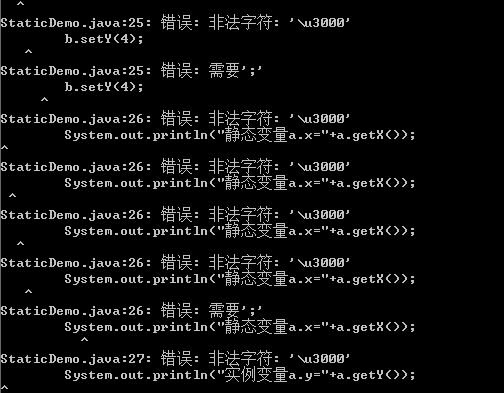java中静态属性、方法的特点。

class StaticDemo
{
int static x=1;
int y=2;
static
{ x=10; }
public int getX()
{
return x;
}
public static void setX(int newX)
{
x = newX;
}
public int getY()
{
return y;
}
public static void setY(int newY)
{
y = newY;
}

public static void main(String[] args) {
System.out.println("静态变量x="+StaticDemo.getX());
StaticDemo a= new StaticDemo();
StaticDemo b= new StaticDemo();
a.setX(1);
a.setY(2);
b.setX(3);
b.setY(4);
System.out.println("静态变量a.x="+a.getX());
System.out.println("实例变量a.y="+a.getY());
System.out.println("静态变量b.x="+b.getX());
System.out.println("实例变量b.y="+b.getY());
}
}2个回答

http://blog.csdn.net/zhandoushi1982/article/details/8453522

System.out.println("静态变量x="+StaticDemo.getX()); 类名.静态方法名字 你的getX是一个非static的